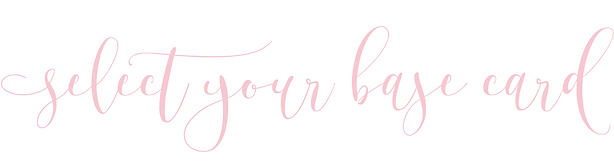## l a s e r - c u t ( p f )## s l i d e - i n## f l a t## s i g n a t u r e (p f)## r e t a n g o l o (p f)## p e r f e t t o (p f )## ve r t i c o ( p f )## p o c k e t f r a m e ( pf )## p a n e l p o c k e t## p e t a l f o l d## a c r y l i c## p o c h e t t e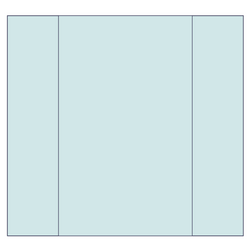## g a t e## f o l d e d## d u p l e x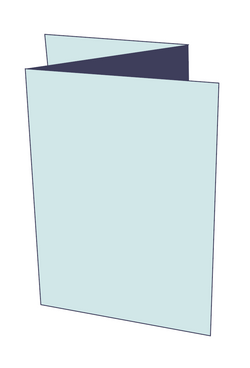## z i g z a g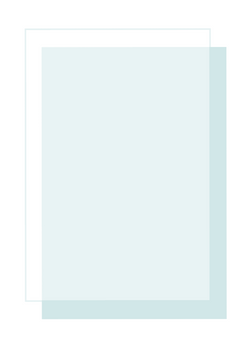## v e l l u m## m a g n e t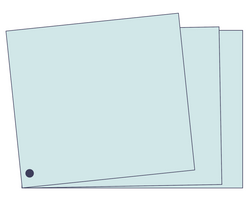## b o o k l e t## p o s t c a r d